# Dynamic Programming vs. Transformation Matrix in Linear Recurrence problems

A linear recurrence problem means a problem that can be represented as a function such that each term is a linear combination of the previous ones. A classical example is Fibonnaci’s: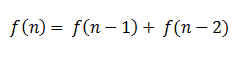Even though this problem can be coded in an iterative way, I’m sure you are agree with me when I say that a recursive one is more elegant:

``````public long fibonacci(int n) {
if (n == 1 || n == 2)
return 1;
return fibonacci(n - 1) + fibonacci(n - 2);
}
``````

However, this first version does not look quite efficient. It is recalculating again and again lot of funcion’s terms. For example, fibonacci(5) needs fibonacci(4) and fibonacci(3). Note that fibonacci(4) also needs fibonacci(3), so it is being calculated again. That’s bad.

To solve that, we can add memoization to store calculated fibonacci(n):

``````public long fibonacci(int n, HashMap<Integer, Long> mem) {
if (n == 1 || n == 2)
return 1;
if (mem.containsKey(n))
return mem.get(n);
long fib = fibonacci(n - 1, mem) + fibonacci(n - 2, mem);
mem.put(n, fib);
return fib;
}
``````

Done! It looks pretty efficient now. In fact, we may think solution above is the most efficient one, but it is not. Keep reading.

Every linear recurrence-based problems can be solved with the next formula: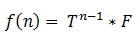Where T is a transformation matrix and F is a column matrix containing the initial values. But, how do we obtain T and F matrices?

First, we need to obtain the value of k. k is defined as the number of previous values needed to obtain the “current one” (i.e. n). In our example, Fibonacci’s, k = 2, because we need f(n-1) and f(n-2) to get f(n); 2 previous values.

We define F as a column matrix where each row is f(1) to f(k):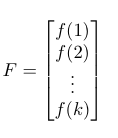In our example, k = 2, f(1) = 1 and f(2) = 1, so: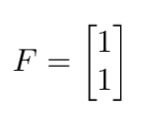Once we have F, we need transformation matrix T. T is a kxk matrix where:

• each row is filled with 0 except the the (i+1)th column, that is 1; where i is the row index.
• last row is composed of the coefficients of each previous term.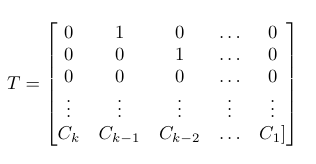Fibonacci expression can be rewritten by: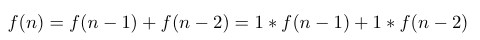Coefficients are 1 and 1, so we define T as: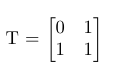We already have all the elements needed to build our formula: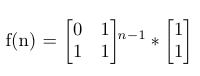Here is a Java implementation:

``````public long fibonacci(int n) {
if (n == 1 || n == 2)
return 1;
long[][] t = {{0, 1}, {1, 1}};
long[][] f = {{1}, {1}};
long[][] tPow = powMatrix(t, n - 1);
return multMatrices2x2and2x1(tPow, f);
}

private long[][] powMatrix(long[][] matrix, int pow) {
if (pow == 1)
return matrix;
long[][] powM = powMatrix(matrix, (int) Math.floor(pow / 2));
if (pow % 2 == 0)
return multMatrices2x2(powM, powM);
return multMatrices2x2(multMatrices2x2(powM, powM), matrix);
}

private long[][] multMatrices2x2(long[][] m1, long[][] m2) {
return new long[][]{
{m1 * m2 + m1 * m2, m1 * m2 + m1 * m2},
{m1 * m2 + m1 * m2, m1 * m2 + m1 * m2}
};
}

private long[][] multMatrices2x2and2x1(long[][] m1, long[][] m2) {
return new long[][]{
{m1 * m2 + m1 * m2},
{m1 * m2 + m1 * m2}
};
}
``````

## Benchmark

After a few tests with both Fibonacci’s versions, here are some numbers: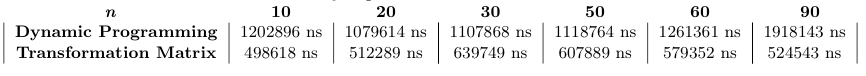It looks like transformation matrix’s version is a bit faster.

Hope you enjoyed the post!

Written on December 4, 2017